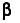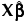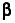Actual by Predicted Plot Plots actual values versus values predicted by the model, but without accounting for the random effects. The Actual by Predicted Plot appears by default. Actual by Predicted Plot Residual Plots Provides residual plots that assess model fit, without accounting for the random effects. Residual Plots Profiler, Contour Profiler, Mixture Profiler, Surface Profiler Provides profilers to examine the relationship between the response and the model terms, without accounting for random effects. Profilers
The Actual by Predicted plot appears by default. It provides a visual assessment of model fit that reflects variation due to random effects. It plots the observed values of Y against the marginal predicted values of Y. These are the predicted values obtained if you select Save Columns > Prediction Formula.
Denote the linear mixed model by E[Y|γ] = Xβ + Zγ. Hereis the vector of fixed effect coefficients and γ is the vector of random effect coefficients. The marginal predictions are the predictions from the fixed effects part of the predictive model, given by.
Denote the linear mixed model by E[Y|γ] = Xβ + Zγ. Hereis the vector of fixed effect coefficients and γ is the vector of random effect coefficients. The marginal residuals are the residuals from the fixed effects part of the predictive model:
Shows the residuals plotted against the predicted values of Y. You typically want to see the residual values scattered randomly about zero.
Denote the linear mixed model by E[Y|γ] = Xβ + Zγ. Hereis the vector of fixed effect coefficients and γ is the vector of random effect coefficients. The marginal predictions are the predictions from the fixed effects part of the predictive model, given by.
 • Profiler
 • Contour Profiler
 • Mixture Profiler
 • Surface Profiler